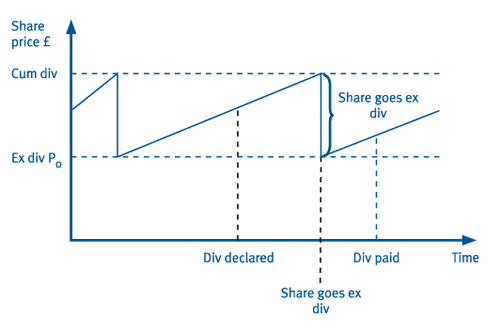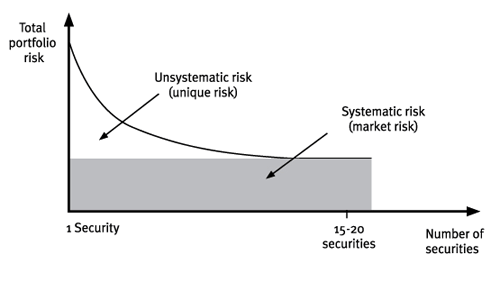# ACCAPEDIA

Kaplan Wiki > ACCAPEDIA > Wiki Pages > Cost of Capital

## # Cost of CapitalFavorite|Discussions
| |History
|### Quick Launch View All Site Content# Cost of capital

Back up a level
 Contents [Hide]

# Cost of capital

An important approach to investment appraisal is to use discounted cash flows. To this requires a discount rate or "cost of capital". This page looks at some of the key ideas in determining a cost of capital that provide the background to specific calculations such as determining the cost of equity or cost of debt.

## Key ideas

### The time value of money

Money received today is worth more than the same sum received in the future, i.e. it has a time value.

This occurs for three reasons:

(DCF) techniques take account of this time value of money when appraising investments.

As a consequence the discount rate used should ideally reflect all three of these:

• potential for earning interest/cost of finance - this approach is emphasised when we use the Dividend VAluation Model (see below) where we "work backwards" to determine the return required by different providers of finance.
• impact of inflation - this will determine whether we want "money" rates or "real" rates. The impact of inflation on discounting is discussed here.
• effect of risk - both CAPM and the use of credit spreads show the link between risk and required return.

### Taxation

Although in the context of equity the company's cost is equal to the investor's required return, the same is not true of debt.

A distinction must thus be made between the required return of debt holders / lenders (Kd) and the company's cost of debt (Kd(1-T)). This is because of the impact of tax relief.

### The weighted average cost of capital (WACC)

Ideally we want a discount rate that reflects the returns of all providers of long term finance.

The WACC is derived by finding a firm's cost of equity and cost of debt and averaging them according to the market value of each source of finance.

One formula for calculating WACC is given  as:• Ve and Vd are the market values of equity and debt respectively.
• ke and kd are the returns required by the equity holders and the debt holders respectively.
• T is the corporation tax rate.
• ke is the cost of equity.
• kd(1-T) is the cost of debt.

Choice of weights

When calculating the WACC the weights may be measured by reference to book values (BVs) or market values (MVs).

Note that when using BVs, reserves such as share premium and retained profits are included in the BV of equity, in addition to the nominal value of share capital.

However, the value of shareholders' equity shown in a set of accounts will often reflect historic asset values, and will not reflect the future prospects of an organisation or the opportunity cost of equity entrusted by shareholders. Consequently it is preferable to use MV weights for the equity.

Note that when using MVs, reserves such as share premium and retained profits are ignored as they are in effect incorporated into the value of equity.

Equally, we should also use the MV rather than the BV of the debt, although the discrepancy between these is likely to be much smaller than the discrepancy between the MV and BVs of equity.

## The Dividend Valuation Model (DVM)

A commonly used method for determining costs of capital is the dividend valuation model (DVM). As the name suggests, it was originally developed for equity but the principles can be adapted for debt and other methods of finance.

### Basic idea

The DVM states that the value of a share can be estimated as the present value of future dividends discounted at the shareholders' required return.

To calculate the shareholders' required return (= the cost of equity) we work backwards:

• The share price = PV of future dividends discounted at the Ke
• If we know the share price and future expectations regarding dividends, then we can deduce the Ke

### Example - dividends with constant growth

If dividends are assumed to grow at a constant rate, g, into perpetuity, then the dividend stream can be discounted to give:

Share price  = PV of growing dividends

P0 = D0(1 + g) / (ke - g)

D 0 = current level of dividend

P 0 = current share price

g = estimated growth rate in dividends

If we need to derive ke the formula can be rearranged to:

ke = [D0 (1+g) / P0 ] + g

### The ex-div share price

The DVM model is based on the perpetuity formula, which assumes that the first payment will arise in one year's time (i.e. at the end of year 1).

Dividends are paid periodically on shares. During the period prior to the payment of dividends, the price rises in anticipation of the payment. At this stage the price is cum div.

Sometime after the dividend is declared the share becomes ex div, and the price drops. This may be expressed diagrammatically:It will be noted that the share goes ex div shortly before the dividend is paid. Any person acquiring the share after this point in time will not receive the dividend, which will be paid to the original shareholder. The reason is that the time it takes for the company to amend its register of members requires a cut-off point somewhat before the dividend is paid.

Thus, when a share is quoted cum div, the price includes both the underlying ex div value of the share (Po), and the dividend due shortly (Do).

In the DVM formula we thus need an ex-div price.

Po represents the 'ex div' share price = Cum div share price - dividend due

Your FeedbackWe value your feedback on the topics or anything else you have found on our site, so we can make it even better.

### Weaknesses of the DVM

Few would argue with the basic premise of the model that the value of a share is the present value of all its future dividends. Its major weakness stems from limitations in the input data.

Current market price

Po - this can be subject to other short-term influences, such as rumoured takeover bids, which considerably distort the estimate of the cost of equity.

Future dividends

For simplicity we usually assume no growth or constant growth.These are unlikely growth patterns. Further, growth estimates based on the past are not always useful; market trends, economic conditions, inflation, etc. need to be considered.

Relevance of earnings in the DVM

Earnings do not feature as such in the DVM. However, earnings should be an indicator of the company's long-term ability to pay dividends and therefore in estimating the rate of growth of future dividends, the rate of growth of the underlying profits must also be considered. For example, if dividends grow at 10% whilst earnings grow at 5%, before long the firm will run out of funds with which to pay dividends. Similarly, if dividends grow at 5% and profits at 10%, the firm will soon accumulate excess funds.

## The Capital Asset Pricing Model (CAPM)

The total return demanded by an investor is actually dependent on two specific factors:

• the prevailing risk-free rate (Rf) of return
• the reward investors demand for the risk they take in advancing funds to the firm.

The Capital Asset Pricing Model (CAPM) gives a way of determining the required return for a given level of risk.

### Systematic and unsystematic risk

An investor, knowing that a particular investment was risky, could decide to reduce the overall risk faced, by acquiring a second share with a different risk profile and so obtain a smoother average return. Reducing the risk in this way is known as diversification.

However risk reduction slows and eventually stops altogether once 15-20 carefully selected investments have been combined.

This is because the total risk faced is not all of the same type.The risk a shareholder faces is in large part due to the volatility of the company's earnings. This volatility can occur because of:

• systematic risk - market wide factors such as the state of the economy
• non-systematic risk - company/industry specific factors.

Systematic risk will affect all companies in the same way (although to varying degrees). Non-systematic risk factors will impact each firm differently, depending on their circumstances.

Diversification can almost eliminate unsystematic risk, but since all investments are affected in the same way by macro-economic i.e. systematic factors, the systematic risk of the portfolio remains.

### The CAPM formula

Required return = Rf + β × (Rm - Rf)

where:

Rf = risk-free rate

Rm = average return on the market

(Rm - Rf) = equity risk premium (sometimes referred to as average market risk premium)

β = systematic risk of the investment compared to market and therefore amount of the premium needed

To calculate a project return the company would need to find a beta that reflects the systematic risk of the project and then use the CAPM equation.

### Well diversified shareholders

Given CAPM only incorporates systematic risk it can only be used for investors (i.e. shareholders) who hold well-diversified portfolios.

CAPM is discussed in more detail here.

## Using the WACC as a discount rate

The WACC calculation is based upon the firm's current costs of equity and debt. It is therefore appropriate for use in investment appraisal provided:

• the historic proportions of debt and equity are not to be changed
• the operating risk of the firm will not be changed
• the finance is not project-specific, i.e. projects are financed from a pool of funds.

or

• the project is small in relation to the company so any changes are insignificant.

If any of these criteria are not met it will not be appropriate to appraise a project using the historic WACC.

A discussion of when the WACC can be used and possible alternative approaches can be found here.Created at 8/21/2012 5:19 PM  by System Account  (GMT) Greenwich Mean Time : Dublin, Edinburgh, Lisbon, London Last modified at 9/13/2013 10:37 AM  by System Account  (GMT) Greenwich Mean Time : Dublin, Edinburgh, Lisbon, London### Rating :Ratings & Comments  (Click the stars to rate the page)### Tags:### Recent Discussions

 There are no items to show in this view.ACCAPEDIA - Cost of Capital